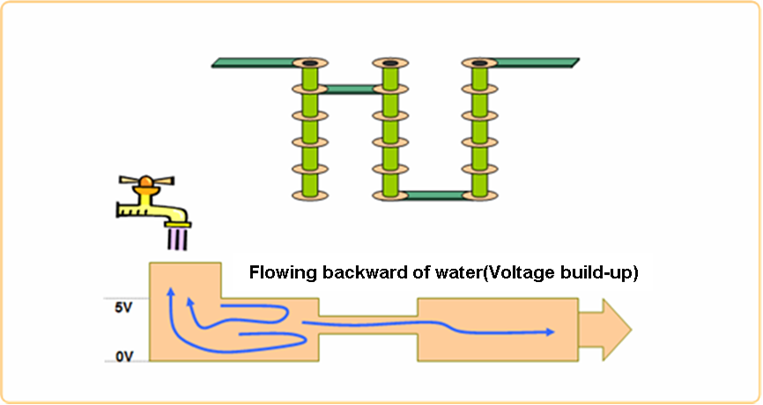# Via Quantity

This item checks layers change by counting the number of Vias used in a signal net.

Some of the signal traces have special characteristics so that they are not allowed to change layers. For the impedance controlling net, layer change is a critical element for impedance shift. In order to prevent impedance shifts, the designer restricts the change of trace width and the change of layers to minimize possibilities of problems.
• Check the number of vias used in signal net

• Item: Input item name.
• Net: Select a target net group.
• Limit: Select via quantify check type between Min and Max.
• Via Qty: Assign the number of vias allowed in a net or nets for the target net group.
• Composite Power Net: DFE uses composited power net instead of single power net. Double-click the item field. The Composite Power Net dialog displays.
• Passive Comp Group: The DFE makes composite net which are connected through this passive component.
• Exception Net Group: Nets that should not be merged into the composite net.
• Start Component: When multiple load components exist, the tool checks via count for each path to load component.
• Check the number of vias used in signal net copper area.
• Via Qty On Copper: Check via quantity of copper area if net has copper area.
• Check pad on via quantity of pin pad which are connected to selected signal net.
• Connected Comp: Select component check pad on via quantity of ground pin pad.
• GND Net: Assign Ground signal group in order to determine GND pin of component.
• Pad on Via Qty(Min): Assign the number of minimum via should be exist on ground pad.
• Composite Power Net: DFE uses composited power net instead of single power net.
• Composite Power Net:
• Passive Comp: The DFE makes composite net which are connected through this passive component.
• Exception Net: Nets which should not be merged into composite net.
In high speed signal, via can make impedance mismatching and result in generating crosstalk. This symptom causes a voltage rise. Therefore, it is recommended to use a limited number of vias.## Capacitance of Vias

${C}_{via}\text{\hspace{0.17em}}=\text{\hspace{0.17em}}\frac{1.41{\epsilon }_{r}T{D}_{1}}{{D}_{2}\text{\hspace{0.17em}}-\text{\hspace{0.17em}}{D}_{1}}$
Where,
${D}_{2}$
Diameter of hole in ground planes, in.
${D}_{1}$
$T$
Thickness of PCB or dielectric, in.
${\epsilon }_{r}$
Relative dielectric constant of PCB material.
$C$
Parasitic via capacitance, pF.
Minimize ${C}_{via}$ by:

## Inductance of Vias

${L}_{via\text{\hspace{0.17em}}}\text{\hspace{0.17em}}=\text{\hspace{0.17em}}5.08h\left[\mathrm{ln}\text{\hspace{0.17em}}\left(\frac{4h}{d}\right)\text{\hspace{0.17em}}+\text{\hspace{0.17em}}1\right]$
Where,
$h$
Length of via, in.
$d$
Diameter of via, in.
$L$
Inductance of via, nH.
Minimize ${L}_{via\text{\hspace{0.17em}}}$ by:
• Eliminating and/or reducing stubs.
• Minimizing via barrel length by routing outer layers near surface layers and applying Back drilling to remove the parasitic stub.